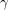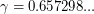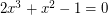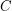# Seymour's Second Neighbourhood Conjecture

 Importance: High ✭✭✭
 Author(s): Seymour, Paul D.
 Subject: Graph Theory » Directed Graphs
 Keywords: Caccetta-Häggkvist neighbourhood second Seymour
 Posted by: nkorppi on: October 9th, 2007
Conjecture   Any oriented graph has a vertex whose outdegree is at most its second outdegree.

By theth outdegree of, we mean the number of vertices for which the minimal outward-directed path fromto them is of length.

Chen, Shen, and Yuster [CSY] proved that in any oriented graph there is a vertex whose second outdegree is at leasttimes its outdegree, whereis the unique real root of.

This conjecture implies a special case of the \Oprefnum[Caccetta-Häggkvist Conjecture]{46385}.

## Bibliography

[ASY] Chen, G.; Shen, J.; Yuster, R. Second neighborhood via first neighborhood in digraphs, Annals of Combinatorics, 7 (2003), 15--20.

[F] Fisher, David C. Squaring a tournament: a proof of Dean's conjecture. J. Graph Theory 23 (1996), no. 1, 43--48.

[KL] Kaneko, Yoshihiro; Locke, Stephen C. The minimum degree approach for Paul Seymour's distance 2 conjecture. Proceedings of the Thirty-second Southeastern International Conference on Combinatorics, Graph Theory and Computing (Baton Rouge, LA, 2001). Congr. Numer. 148 (2001), 201--206.

* indicates original appearance(s) of problem.

### It is proved

This conjecture has been proved. You can find the proof here .

### Re: It is proved

Thanks for the reference. The proof, however, seems flawed. The basic outline of the proof (if I understood it correctly) is as follows:

\item Ifhas a sink, then this sink satisfies the conditions. \item An oriented graph without directed cycles has a sink. \item Then one proves directly that a graph containing a directed cycle has a vertex (even on that cycle) that satisfies the conditions.

The last part (Lemma 2 of the manuscript), is not true -- take a directed cycle, add many independent points, and add all the arcs fromto. Now the conjecture is true for this graph (one can take any of the sinks -- vertices in), but it is not true that one can choose a vertex of.

The omission in the proof of Lemma 2 is that it's tacitly assumed, that adjacent vertices of the cycle have no common out-neighbours.

### So is this proof valid or

So is this proof valid or not? I am currently working on a proof and am wondering whether or not I should be bothering

### Re: So is this proof valid or

I believe the mentioned prof is not valid. Is your proof working? And sorry for the late reply, our system falsely recognized your comment as a spam.

### Counterexample for the proof

As was indicated, Lemma 2 is false. Here is a counterexample to lemma 2. Note that it is not a counterexample to the conjecture, since it has two sinks.